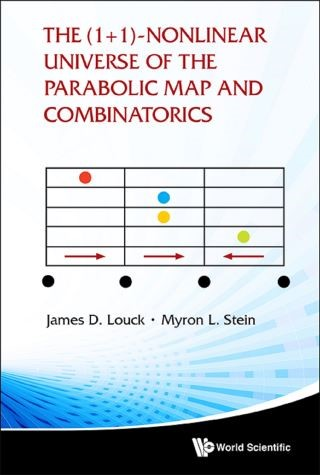## (1+ 1)-nonlinear Universe Of The Parabolic Map And Combinatorics, The• Description
• Author
• Info
• Reviews

### Description

This monograph develops chaos theory from properties of the graphs inverse to the parabolic map of the interval [0, 2], where the height at the midpoint x = 1 may be viewed as a time-like parameter, which together with the x-coordinate, provide the two parameters that uniquely characterize the parabola, and which are used throughout the monograph. There is only one basic mathematical operation used: function composition. The functions studied are the n-fold composition of the basic parabola with itself. However, it is the properties of the graph inverse to this n-fold composition that are the objects whose properties are developed. The reflection symmetry of the basic parabola through the vertical line x = 1 gives rise to two symmetry classes of inverse graphs: the inverse graphs and their conjugates. Quite remarkably, it turns out that there exists, among all the inverse graphs and their conjugates, a completely deterministic class of inverse graphs and their conjugates. Deterministic in the sense that this class is uniquely determined for all values of the time-like parameter and the x-coordinate, the entire theory, of course, being highly nonlinear — it is polynomial in the time-like parameter and in the x-coordinate. The deterministic property and its implementation are key to the argument that the system is a complex adaptive system in the sense that a few axioms lead to structures of unexpected richness.

This monograph is about working out the many details that advance the notion that deterministic chaos theory, as realized by a complex adaptive system, is indeed a new body of mathematics that enriches our understanding of the world around us. But now the imagination is also opened to the possibility that the real universe is a complex adaptive system.

* deceased

Contents:
• Introduction and Point of View
• Recursive Construction
• Description of Events in the Inverse Graph
• The (1+1)-Dimensional Nonlinear Universe
• The Creation Table
• Graphical Presentation of MSS Roots
• Graphical Presentation of Inverse Graphs

Readership: Post-graduates from mathematics and physics backgrounds, mathematics and physics professionals with an interest in astrophysics.
Key Features:
• There exists a unique inverse graph. This unique graph can be constructed from a simple algorithm
• The concept of a deterministic inverse graph and its relationship to complex adaptive systems is new
• This monograph is of general value to readers because it illustrates nicely that research in new methodologies in Mathematics can lead to new insights in Physics